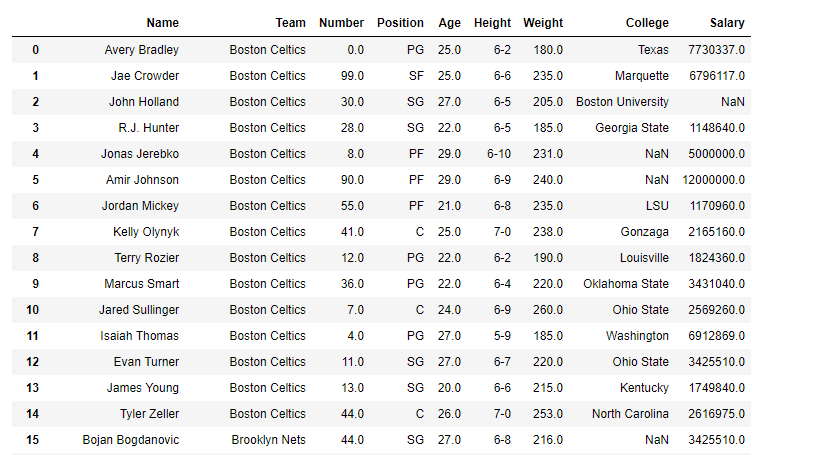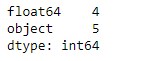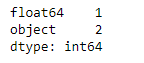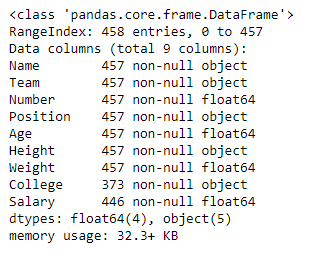Related Articles
Python | Pandas dataframe.get_dtype_counts()
• Last Updated : 19 Nov, 2018

Python is a great language for doing data analysis, primarily because of the fantastic ecosystem of data-centric python packages. Pandas is one of those packages and makes importing and analyzing data much easier.

Pandas` dataframe.get_dtype_counts()` function returns the counts of dtypes in the given object. It returns a pandas series object containing the counts of all data types present in the pandas object. It works with pandas series as well as dataframe.

Syntax: DataFrame.get_dtype_counts()

Returns : value : Series : Counts of datatypes

Example #1: Use `get_dtype_counts()` function to find the counts of datatype of a pandas dataframe object.

 `# importing pandas as pd ` `import` `pandas as pd ` ` `  `# Creating the dataframe  ` `df ``=` `pd.read_csv(``"nba.csv"``) ` ` `  `# Print the dataframe ` `df `Now apply the `get_dtype_counts()` function. Find out the frequency of occurrence of each data type in the dataframe.

 `# applying get_dtype_counts() function  ` `df.get_dtype_counts() `

Output :Notice, the output is a pandas series object containing the count of each data types in the dataframe.

Example #2: Use `get_dtype_counts()` function over a selected no. of columns of the data frame only.

 `# importing pandas as pd ` `import` `pandas as pd ` ` `  `# Creating the dataframe  ` `df ``=` `pd.read_csv(``"nba.csv"``) ` ` `  `# Applying get_dtype_counts() function to  ` `# find the data type counts in modified dataframe. ` `df[[``"Salary"``, ``"Name"``, ``"Team"``]].get_dtype_counts() `Notice, the output is a pandas series object containing the count of each data types in the dataframe. We can verify all these results using this the `dataframe.info()` function.

 `# Find out the types of all columns in the dataframe ` `df.info() `

Output :Attention geek! Strengthen your foundations with the Python Programming Foundation Course and learn the basics.

To begin with, your interview preparations Enhance your Data Structures concepts with the Python DS Course.

My Personal Notes arrow_drop_up
Recommended Articles
Page :